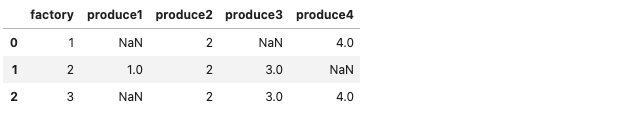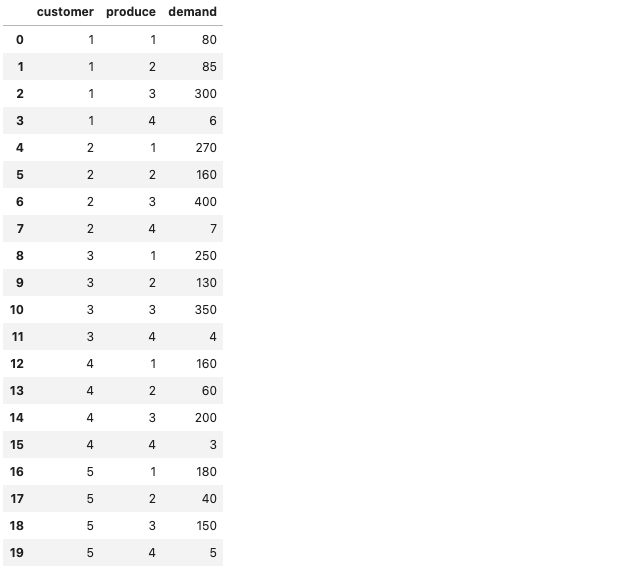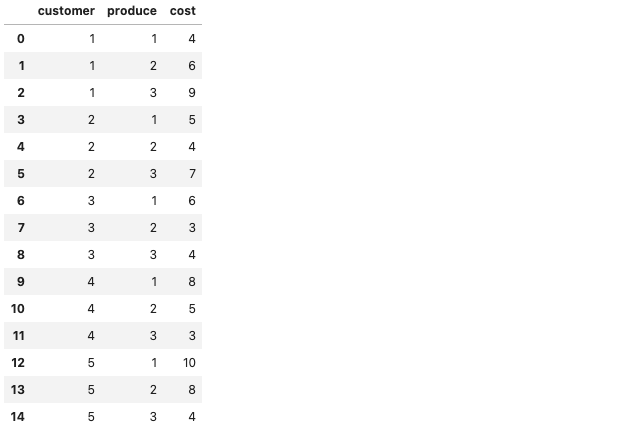# [PYTHON] [Memo] I implemented a multi-product transportation problem (new mathematical optimization)

• Articles sent by data scientists from the manufacturing industry
• This time, I bought a book of New Mathematical Optimization, so I arranged and implemented the parts that could be used in practice.

## Introduction

Since I have more opportunities to use optimization in my work, I bought a book called "New Mathematical Optimization", so I am studying to use it in practice. If you want to know more details, please purchase the book as there may be a problem if you include the details.

## Implementation of high-mix transportation problem

This time we have implemented a shipping issue to carry multiple products. The library I used was mypulp. Although gurobi is used in the book, I chose mypulp which can be used for free because it is charged. When implementing mathematical optimization in python, I think that pulp is often used, but since it is written in a slightly different way from gurobi, I have selected mypulp that can be implemented in the same way as gurobi.

Also, when using it in practice, I thought that it is common to read data such as constraints with csv and use it, so I have partially modified it.

The implementation code of python is below.

``````#Load the required libraries
import pandas as pd
from mypulp import *
``````

Next, I will write the code to solve the multi-product transportation problem. The constraints and objective functions are described below.

``````def mctransp(I, J, K, c, d, M):
"""Multi-product transportation problem

Arg:
I(set)  :Customer number
J(list) :Factory number
K(list) :Product No
c(dict) : Key:(Customer number,Factory number,Product No), Value:Shipping costs
d(dict) : Key:(Customer number,Product No), Value:Demand
M(dict) : Key:Factory number, Value:Production capacity

Returns:
a model, ready to be solved.
"""
#Model definition
model = Model(name = "Multi-product_transportation_problem")

#Create dictionary x to store variables
#Variables are generated only if there is a key in dictionary c that represents shipping costs
x = {}
for i,j,k in c:
model.update()

arcs = tuplelist([(i,j,k) for (i,j,k) in x])

#Customer demand constraints
for i in I:
for k in K:
model.addConstr(quicksum(x[i,j,k] for (i,j,k) in arcs.select(i,"*",k)) == d[i,k])

#Factory capacity constraints
for j in J:
model.addConstr(quicksum(x[i,j,k] for (i,j,k) in arcs.select("*",j,"*")) <= M[j])

#Objective function
model.setObjective(quicksum(c[i,j,k]*x[i,j,k]  for (i,j,k) in x), GRB.MINIMIZE)

model.update()
model.__data = x
return model
``````

The following is a function to get each condition. In the book, it was hard-coded, but I thought it would be easier to use if I changed to the method of reading from the csv file, so I arranged it a little.

``````def get_data():
"""Input data acquisition

Return:
I(set)  :Customer number
J(list) :Factory number
K(list) :Product No
c(dict) : Key:(Customer number,Factory number,Product No), Value:Shipping costs
d(dict) : Key:(Customer number,Product No), Value:Demand
M(dict) : Key:Factory number, Value:Production capacity
"""
#Extract products that can be manufactured at the factory
#Convert DataFrame to dict
produce = df_p.set_index('factory').T.to_dict('list')
#Remove Nan
for key in produce.keys():
produce[key] = {x for x in produce[key] if x==x}

#Extract customer and product demand
#Create a tuple of customer number and product number
D = list(zip(df_d[df_d.columns], df_d[df_d.columns]))
#Create a dictionary with the amount of demand as the key, using the tuple (pair) of the customer number and product number as the key.
d = dict(zip(D, df_d[df_d.columns]))

#Generate customer number I
I = set([i for (i,k) in d])
#Create factory number list J and production capacity M using multidict
J, M = multidict({1:3000, 2:3000, 3:3000})
#Create product number list K and weight weight using multidict
K, weight = multidict({1:5, 2:2, 3:3, 4:4})

#Extract shipping costs for customers and goods
#Create a tuple of customer number and product number
C = list(zip(df_c[df_c.columns], df_c[df_c.columns]))
#Create a dictionary with shipping costs as the key, using tuples (tuples) of customer numbers and product numbers as keys.
cost = dict(zip(C, df_c[df_c.columns]))

#Calculate the shipping cost for each product from weight and cost and store it in dictionary c
c = {}
for i in I:
for j in J:
for k in produce[j]:
c[i, j, k] = cost[i, j] * weight[k]

return I, J, K, c, d, M
``````

Finally, solve the optimization.

``````if __name__ == "__main__":
I, J, K, c, d, M = get_data()
model = mctransp(I, J, K, c, d, M)
#Perform optimization
model.optimize()
print("Opt value:", model.ObjVal)

# Opt value: 43536.0
``````

The csv file used for reference is described.

``````#Extract products that can be manufactured at the factory
#Convert DataFrame to dict
produce = df_p.set_index('factory').T.to_dict('list')
#Remove Nan
for key in produce.keys():
produce[key] = {x for x in produce[key] if x==x}

# produce
# {1: {2.0, 4.0}, 2: {1.0, 2.0, 3.0}, 3: {2.0, 3.0, 4.0}}
````````````#Extract customer and product demand
#Create a tuple of customer number and product number
D = list(zip(df_d[df_d.columns], df_d[df_d.columns]))
#Create a dictionary with the amount of demand as the key, using the tuple (pair) of the customer number and product number as the key.
d = dict(zip(D, df_d[df_d.columns]))

# d
# d = {(1,1):80,   (1,2):85,   (1,3):300, (1,4):6,
#      (2,1):270,  (2,2):160,  (2,3):400, (2,4):7,
#      (3,1):250,  (3,2):130,  (3,3):350, (3,4):4,
#      (4,1):160,  (4,2):60,   (4,3):200, (4,4):3,
#      (5,1):180,  (5,2):40,   (5,3):150, (5,4):5
#     }
````````````#Extract shipping costs for customers and goods
#Create a tuple of customer number and product number
C = list(zip(df_c[df_c.columns], df_c[df_c.columns]))
#Create a dictionary with shipping costs as the key, using tuples (tuples) of customer numbers and product numbers as keys.
cost = dict(zip(C, df_c[df_c.columns]))

# cost
# cost = {(1,1):4,  (1,2):6,  (1,3):9,
#         (2,1):5,  (2,2):4,  (2,3):7,
#         (3,1):6,  (3,2):3,  (3,3):4,
#         (4,1):8,  (4,2):5,  (4,3):3,
#         (5,1):10, (5,2):8,  (5,3):4,
#        }
``````## at the end

Thank you for reading to the end. Since optimization is often required at the manufacturing site, I will continue to study mathematical optimization.

If you have a request for correction, we would appreciate it if you could contact us.